# Introduction to matrix mathematics

4.3In this chapter, we’re going to be taking a closer look at matrices. Now, this is a mathematical concept by nature. And in the next chapter, you’ll see how it ties in with finance. For now, it’s best to leave the investment and finance at the door and put on your math thinking cap. With that done, let’s get started with matrices.

### What is a matrix?

A matrix is a rectangular array of numbers or expressions. These numbers or expressions are arranged in rows and columns. And they are contained within boxed/square brackets. Here are the other key details about matrices.

• The numbers or expressions in a matrix are called elements.
• The horizontal line entries in a matrix are called rows.
• The vertical line entries are called columns.
• The size of a matrix is represented using its rows and columns. So, a matrix with p rows and q columns is called a ‘p x q matrix.’ This is read as a ‘p by q matrix.’

Let’s take a look at a matrix to understand this better. We’ll call this matrix M.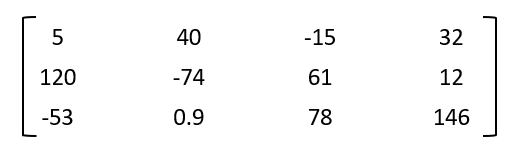What can you gather from this matrix?

• Here, there are 3 rows and 4 columns.
• So, this is a 3 x 4 matrix - read as a 3-by-4 matrix.
• The elements in a matrix can be positive, negative, fractions, decimals, or just about anything.

There is another generic way to represent these elements in matrix M too.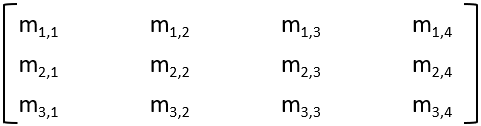Basically, the first number represents the row number and the second represents the column number.

### Transpose of a matrix

The transpose of a matrix is formed by turning its rows into its columns, and its columns into its rows. So, if you originally have a 3 x 4 matrix, its transpose will be a 4 x 3 matrix. The transpose of a matrix M is represented as MT. Similarly, the transpose of a matrix A is represented as AT.

Let’s take up the same matrix we saw earlier and check out what its transpose looks like.

 This is the original matrix M.And this is the transpose of it. (Matrix MT)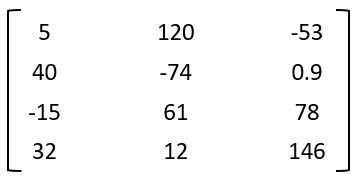Now, here’s where it gets interesting. You can perform addition and subtraction using matrices. Here are the basic rules for adding two matrices.

• The two matrices should be of the same size. You cannot add two matrices with different dimensions. So, you can add a 2 x 2 matrix and another 2 x 2 matrix. However, you cannot add a 2 x 2 matrix and a 2 x 3 matrix, or a 2 x 2 matrix and a 4 x 3 matrix. You get the idea, right?
• Secondly, when adding two matrices, you add the individual elements in one matrix with the individual elements in the other matrix. This means you add the element in row 1, column 1 of the first matrix with the element in row 1, column 1 of the second matrix. And the element in row 1, column 2 of the first matrix with the element in row 1, column 2 of the second matrix. And so on.

Let’s take a couple of 2 x 2 matrices to make this procedure clearer.

 This is matrix A.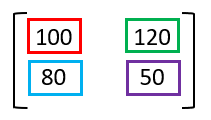This is matrix B.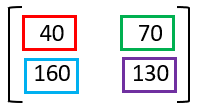See the boxes with different colors? Those are the numbers we’ll be adding. So, the new matrix formed by adding A and B will be as explained below.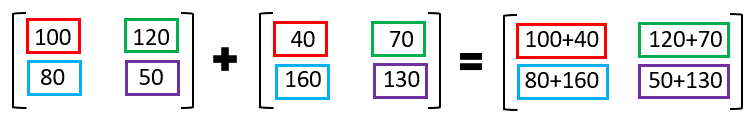The new matrix is: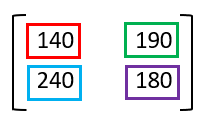Subtraction works just like this, except that the elements are subtracted from one another instead of being added.

### Matrix multiplication

Matrix multiplication is a tad bit more complex. First, we’ll look at scalar multiplication, which is basically just multiplying a matrix with a whole number. For instance, say you have a matrix called matrix M. Now, if you want to multiply this matrix by 2, you will simply multiply each element in the array by 2. Similarly, to multiply a matrix by, say 17, you will simply multiply each element in the array by 17.

Here’s an example.

This is matrix M.

 12 14 19 54 60 17

To multiply this matrix by 4, you will need to multiply each element in the array by 4. Then, this is what we get as the product for the operation Matrix M x 4:

 48 56 76 216 240 68

You can also multiply two matrices. But this is only possible if the number of columns in the first matrix is equal to the number of the rows in the second matrix.

• So, you can multiply a p x q matrix with a q x r matrix.
• Here, the number of columns in the first matrix (q) is equal to the number of rows in the second matrix (also q).
• The size of the new matrix will be p x r.
• To multiply the matrices, each element in the rows of the first matrix is multiplied with the elements in the columns of the second matrix. These products are then added to arrive at the elements in the new matrix.

That was a bit confusing, right? Don’t worry. We’ll look at an example to make it easier. Take these two matrices:

• Matrix A, which is a 3 x 2 matrix
• Matrix B, which is a 2 x 3 matrix

Matrix A

Matrix B

 a1,1 a1,2 a2,1 a2,2 a3,1 a3,2

 b1,1 b1,2 b1,3 b2,1 b2,2 b2,3

Multiplying this 3 x 2 matrix A with the 2 x 3 matrix B will give us a new 3 x 3 matrix C. This is how the elements of that new matrix are formed.

Matrix A x Matrix B = Matrix C

 (a1,1 x b1,1) + (a1,2 x b2,1) (a1,1 x b1,2) + (a1,2 x b2,2) (a1,1 x b1,3) + (a1,2 x b2,3) (a2,1 x b1,1) + (a2,2 x b2,1) (a2,1 x b1,2) + (a2,2 x b2,2) (a2,1 x b1,3) + (a2,2 x b2,3) (a3,1 x b1,1) + (a3,2 x b2,1) (a3,1 x b1,2) + (a3,2 x b2,2) (a3,1 x b1,3) + (a3,2 x b2,3)

We’ll now take up two such matrices with numbers to make the multiplication clearer.

Here, we’ll again take a 3 x 2 matrix and a 2 x 3 matrix.

Matrix A

Matrix B

 2 3 4 5 6 7

 10 20 30 40 50 60

Multiplying these two matrices, here’s what we get:

Matrix A x Matrix B = Matrix C

 (2 x 10) + (3 x 40) (2 x 20) + (3 x 50) (2 x 30) + (3 x 60) (4 x 10) + (5 x 40) (4 x 20) + (5 x 50) (4 x 30) + (5 x 60) (6 x 10) + (7 x 40) (6 x 20) + (7 x 50) (6 x 30) + (7 x 60)

So, matrix C becomes:

 140 190 240 240 330 420 340 470 600

#### Wrapping up

The reason we went into the details of matrices is because when you’re calculating the variance and the risk of a portfolio, you are essentially dealing with matrices. Let’s take a look at the image from the previous chapter, where we had listed the prices of two stocks - A and B - over a 10-day period.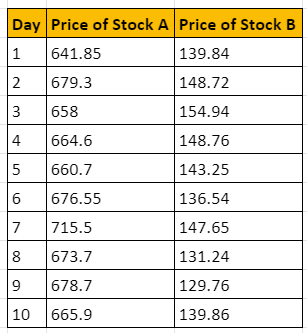See how the prices for a 10 x 2 matrix? Similarly, when you calculate the daily returns for the two stocks, another matrix is formed. So, we’re inherently dealing with these kinds of arrays here. Now, head to the next chapter to discover how it all falls into place.

#### A quick recap

• A matrix is a rectangular array of numbers or expressions. These numbers or expressions are arranged in rows and columns.
• The size of a matrix is represented using its rows and columns. So, a matrix with p rows and q columns is called a ‘p x q matrix.’ This is read as a ‘p by q matrix.’
• The transpose of a matrix, represented as MT, is formed by turning its rows into its columns, and its columns into its rows. So, if you originally have a 3 x 4 matrix, its transpose will be a 4 x 3 matrix.
• You can perform addition and subtraction using matrices. For this, the two matrices should be of the same size. You cannot add two matrices with different dimensions.
• You can also multiply two matrices. But this is only possible if the number of columns in the first matrix is equal to the number of the rows in the second matrix.